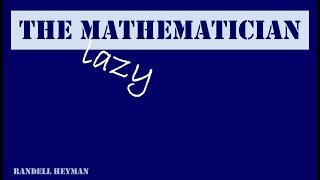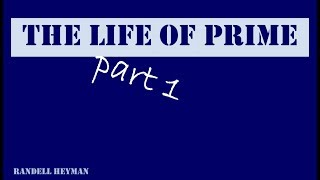## Randell HeymanRandell Heyman

## Work and education

Doctor degree
University of New South Wales

## User videosThe Lazy Mathematician
Sometimes a lazy mathematician can be a good mathematician. In the process we learn about modular arithmetic.The Life of Prime - Part 1
For thousands of years prime numbers were beautiful, interesting .....but useless. If you understand modular arithmetic explains the mathematics of RSA public code encryption. Additional tags: randal randall hayman rsa code prime primes pure mathematics mathematical stories public key encryption